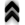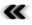### 6.3 Topology

From among the diﬀerent qualiﬁcations resulting from the combination of a set and a relation, the concept of a partially ordered set (poset) is of special importance for the implementation of concepts relating to topology (Deﬁnition 28). Where the topology of the underlying structure cannot be changed, it can be employed to model diﬀerent topologies on top of it. The topological spaces required for manifolds (Deﬁnition 35) as well as ﬁber bundles (Deﬁnition 40) are of particular interest in this context. They can be represented using a collection of connected cells forming a CW complex (Deﬁnition 34).

As topology is constructed on the foundations of set theory, it is only natural that the speciﬁcation of topological structures retains a semblance to the structures of sets. However, since topology requires as well as provides additional information, the interface must accommodate this fact. Here the most prominent requirements are dimension and global space properties.

The GSSE code snippet in Section 6.1 already presents the deﬁnition of a homogeneous interface to a set. Here, topological spaces are now introduced by using the same GSSE data structure speciﬁcation as used before. So far diﬀerent data structures and higher abstraction always required to introduce new types. These types mostly demanded further code adaptation, e.g., diﬀerent data containers and hence iteration modiﬁcations. By using GSSE speciﬁcations, all data structure and topological properties remain consistent and no traversal modiﬁcation is necessary. The important diﬀerence here is that data structures are not restricted to 0D cell types, but can instantiate arbitrary-dimensional CW complex spaces, at compile time and run time.

: Example explicit 2D simplex mesh structure.
typedef gsse::map<
gsse::pair<tag_dimension, mpl::int_<2> >
, gsse::pair<tag_cell,      gsse::cell_simplex >
, gsse::pair<tag_complex,   gsse::complex_explicit >
> cw_complex_t;

The given tags instantiate a 2D simplex cell complex, where the internal cell structure (cell topology) is calculated at compile time and available at run time without performance losses. Arbitrary explicit and implicit cell calculation algorithms can be implemented and used.

Also the implicit and explicit nature of polynomials, given in Section 6.2, can be found in this data type deﬁnitions, where computer storage capacities are formed by implicit and explicit declarations.

: Example implicit 4D hypercube grid structure.
typedef gsse::map<
gsse::pair<tag_dimension, mpl::int_<4> >
, gsse::pair<tag_cell,      gsse::cell_hcube >
, gsse::pair<tag_complex,   gsse::complex_implicit >
> cw_complex_t;

Implicit higher-dimensional topological structures can be identiﬁed by dense grids, where no explicit cell indices are stored in memory. Instead all necessary grid topology is calculated on demand.

As introduced in Section 4.2 algebraic concepts enable operators on structures. Using a mapping between two algebraic structures, cell boundaries can be easily introduced. These operators and mappings beneﬁt from the abstract topological speciﬁcation and operate on spaces of arbitrary dimension and topology.

Given the 4D hypercube structure, the boundary from the boundary, a quadrilateral-on-cell operation, is obtained by the following piece of code, which allows for the calculation of the boundary at compile time:

typedef
gsse::boundary<4, 2, gsse::cell_hcube>
boundary_t;

To allow for such a concise formulation of the task of boundary extraction, several supporting constructs need to be implemented, as was illustrated in the example shown in Section 3.3. At the core of the shown boundary operation is the availability of detailed information regarding the cell’s structure. This structure can be made available using hard coded look up tables; this, however, results in a loss of ﬂexibility, as new look up tables need to be incorporated manually. Alternatively, it may be possible to determine the structure algorithmically. Any such algorithm naturally needs to take into account the type of the cell (e.g., if it is a cuboid or simplex cell). Since the combinatorical problem of determining all structural components increases dramatically  with dimension, a run time algorithm may become prohibitively expensive in case of high performance applications. Therefore, the availability of an algorithm at compile time which can accommodate a change of dimensionality without the need to manually provide and adjust static tables is desirable, even at the expense of increased compile times.

Where boundary operations are essential for numerical schemes employed for the solution of partial diﬀerential equations, the extraction of iso-surfaces is an invaluable tool for the processing of results obtained from any such calculations.

Marching cubes  has been a key algorithm for the rapid extraction of surfaces. It however not only has issues with topological ambiguity, but has also been handicapped by patenting issues [? ]. Both of these issues have been addressed by the development of the marching tetrahedra , which suﬀers neither patent issues nor topological ambiguity.

The following presents a means utilizing generic programming methods and paradigms, which allows to forgo the use of a hard coded look-up table and instead utilize higher level representations of the underlying topological information or determine a look-up table as needed. The procedure is realized using the capabilities already present in the GSSE In this fashion the procedure is generalized to arbitrary dimensions. This allows to supply an algorithm for surface extraction which is suitable for data of any supplied dimension, since it will adapt to the supplied dimension.

The original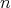-cube cell is decomposed into simplices. It is easy to obtain all the edges from the simplices generated in this fashion. The edges are essential for the surface extraction process, since the vertices which are subsequently used to spawn the extracted surface representation are derived directly from them, by means of interpolation. While it may be tempting to discard the simplex information, since vertex generation is so tightly coupled to the edges, the simplices are essential for the generation of surface elements as they eliminate ambiguity. For, while the generation of surface elements within any given simplex can be done consistently, the lone set of vertices in an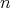-cube leaves too many degrees of freedom undetermined, which is also the source for ambiguity, when using the marching cubes algorithm . While this situation is easily containable in the three-dimensional case, the eﬀort required in higher dimensions increases dramatically .

The task of determining the simplices ﬁlling an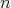-cube does not have a unique solution, as is already apparent in the case of a three-dimensional cube, which admits a ﬁlling consisting of either ﬁve or six tetrahedra. While the generation of a minimal number of elements is desirable, the minimization of the element count is not a driving motivation, but symmetry needs to be considered in order to enable a seamless repetition of neighbouring cells. In the particular case of the three-dimensional cube this condition singles out the decomposition into six tetrahedra. In more general terms the composition in which all tetrahedra share the space diagonal which crosses the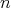-cube cell meets the required symmetry conditions.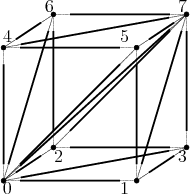Figure 6.1: Vertices forming a 3-cube. All of the shown edges belong to tetrahedra obtained by the described algoirthm.

The proposed algorithm utilizes information available from the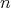-cube. When utilizing the topological mechanisms available in the GSSE, very detailed information regarding the arrangement of lower dimensional components is already available. The proposed algorithm therefore reuses as much of this information as possible.

Starting with an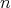-cube cell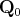; ﬁrst the space diagonal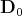is extracted and its vertices stored for simplex construction, before the boundary elements of the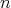-cube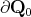, which are themselves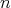-cubesagain, are queried. From each of the boundary elements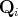, which are of course of lower dimension than before,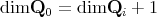, the space diagonal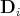is extracted and its vertices added to the simplex again before recursing to once more determining the boundary elements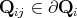of the current cell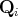. The procedure is applied recursively, until the cells are themselves edges and thus cannot support a distinct diagonal. The space diagonals, thusly generated in conjunction with the edge boundary of the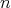-cube form the complete set of edges required to extract a surface representation from the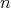-cube. Simplices are determined while selecting the space diagonals and aggregating the encountered vertices.

An example using a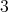-cube is provided to further illustrate the procedure of the algorithm. Considering an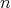-cube cell formed by vertices as illustrated in Figure 6.1. The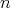-cube cell is given as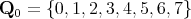. The ﬁrst step is to extract the diagonal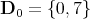. The vertices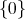and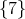are two initial vertices of all the tetrahedra, which will be constructed, thus this edge is shared among all the generated tetrahedra. The boundary elements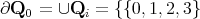,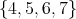,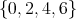,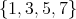,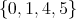,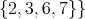are then each considered in turn, giving the diagonals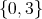,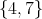,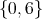,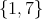,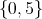,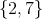, respectively. As can be seen every one of the additional diagonals contains a new vertex to build a tetrahedron, when comparing to the already selected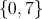vertices. Adding this vertex the list of partial simplices is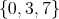,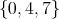,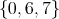,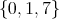,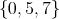,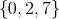, which are now missing a single vertex for completion. As no further diagonals can be generated no further recursion is initiated. However, the vertices of the edges are examined to complete the forming simplices. This ﬁnally gives the tetrahedra to be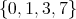,,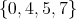,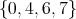,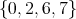,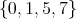.

The simplices are traversed by decomposing them into their edge components. Thereby the membership relations between the simplices and the edges are available naturally. The overall complexity of the algorithm is broken down due to the reuse of the GSSE’s topological operations. However, edges may be evaluated several times, as they belong to several diﬀerent simplices, which comes as overhead if left unaddressed.

As each of the generated simplices is traversed the vertices originating from the edges are used for the formation of surface elements immediately, thus once all simplices have been traversed the surface has been extracted.

The problem of splitting an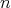-cube into simplices is relatively expensive in terms of computational eﬀort and grows considerable as dimension increases. It is therefore prudent to reuse the extracted topological structures as much as possible. Fortunately, the topological decomposition of an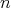-cube into simplices is required to be performed only once and can be reused multiple times once the information has been initialized. Furthermore, traversal of the individual simplices for every cell can be omitted, when the information is condensed into an associative container, which mimics the hard coded look-up tables already in use. However, instead of only guiding subsequent formation of elements one by one, the generic approach may be used to provide all of the resulting surface elements in its own container. Since the GSSE can provide topological information it is feasible to implement the entire algorithm using meta programming techniques .

Thus it is possible to concisely access a surface extraction in the following manner:

tempalte<typename InputDomainType, typename SurfaceDomainType,
typename ParameterPackageType>
void surface_extractor(InputDomainType& input_domain,
SurfaceDomainType& output_surface,
const ParameterPackageType& parameters)
{
typedef typename get_cell_type<InputDomainType>::type
cell_type;
typedef typename get_cell_type<SurfaceDomainType>::type
sim_type;
marching_simplices<cell_type, sim_type,
ParameterPackageType>::type
march(parameters);

gsse::traverse<at_cl>
[
insert(output_surface, march(_1) )
] ( domain ) ;
}

It utilizes the functional paradigm in the spirit of Boost Phoenix  which is enabled by GSSE facilities for traversal and extends the GSSE by a surface extraction mechanism. The parameter pack can be used to supply a prescription for interpolation, so as to provide an additional degree of adaptivity.

template<typename ContainerType>
struct generator
{
ContainerType return_template;
template<typename InitialisationType>
generator(InitialisationType
{  ...  }
{  ...  }
};

The InitialisationType encodes the instructions to initialize the return_template at construction time. Subsequent calls to the operator() use this information to assemble and return the correct surface elements by remapping the representation, which is local to the cell, to the global indices. By initializing the member variable in this fashion in a templated constructor, where the type is utilized for control enables the structs to share the same type and thus be easily stored in the associative container, while at the same time returning diﬀerent values at evaluation time.

Since it is not possible to invoke the templated constructor explicitly, the compiler needs to deduce the type automatically, a dummy pointer argument is used, whereby any instantiation of the passed type can be avoided by using

generator( (type) 0 )

For compatibility with the containers in the STL, a default constructor is also required.

It should go unnoted that the size of the associative container increases quickly with increasing dimension, if symmetry is not utilized to reduce the number of diﬀerent cases. This can be accomplished by establishing a canonical ordering which is then mapped to the rest by permutations corresponding to the symmetries.